# Transformations and Angle Relationships

## Objective

Find missing side lengths in similar figures. Find scale factor between similar figures.

## Common Core Standards

### Core Standards

?

• 8.G.A.4 — Understand that a two-dimensional figure is similar to another if the second can be obtained from the first by a sequence of rotations, reflections, translations, and dilations; given two similar two-dimensional figures, describe a sequence that exhibits the similarity between them.

?

• 7.G.A.1

• 7.RP.A.2

• 7.RP.A.3

## Criteria for Success

?

1. Use ratio reasoning between corresponding sides in similar figures to determine missing measurements.
2. Find the scale factor between similar figures and use it to find missing measurements.
3. Determine if two figures are similar by testing for a scale factor between the side lengths.

## Tips for Teachers

?

In this lesson, students apply the concept of scale factor to determine if shapes are similar with proportional corresponding sides.

#### Fishtank Plus

• Problem Set
• Student Handout Editor
• Vocabulary Package

## Anchor Problems

?

### Problem 1

Two triangles are shown below. The measure of angle $A$ is equal to the measure of angle $D$. (Figures are not drawn to scale.)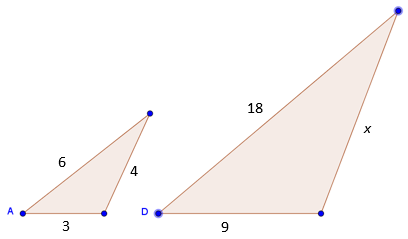1. Explain, using transformations, how you know the two triangles are similar.
2. Find the value for the side length marked $x$.

### Problem 2

Are triangles ${{ABC}}$ and ${{EFG}}$ similar? Explain your answer.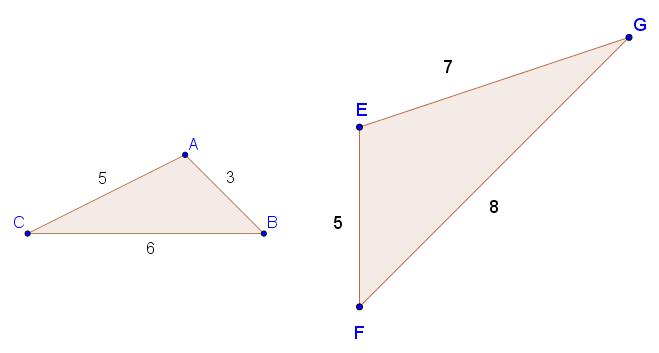Sketch a triangle that is similar to either triangle ${{ABC}}$ or triangle ${{EFG}}$. Label the side lengths.

### Problem 3

Triangle ${{ABC}}$ is similar to triangle ${{ADE}}$. The measure of ${\overline{AB}}$ is approximately ${3.16}$.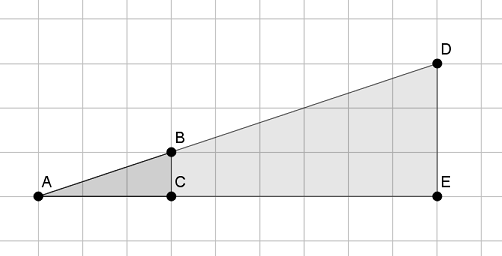1. Find the scale factor from triangle ${{ABC}}$ to ${{ADE}}$.
2. Find the approximate measure of ${\overline{AD}}$.

## Problem Set

?The following resources include problems and activities aligned to the objective of the lesson that can be used to create your own problem set.

?

### Problem 1

In the diagram below, you have triangle ${ABC}$ and triangle ${A'B'C'}$. Based on the information given, are the two triangles similar? Explain your answer.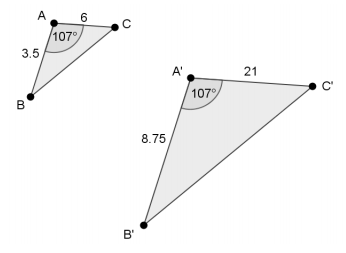#### References

EngageNY Mathematics Grade 8 Mathematics > Module 3 > Topic B > Lesson 11Teacher Version: Exit Ticket

Grade 8 Mathematics > Module 3 > Topic B > Lesson 11 of the New York State Common Core Mathematics Curriculum from EngageNY and Great Minds. © 2015 Great Minds. Licensed by EngageNY of the New York State Education Department under the CC BY-NC-SA 3.0 US license. Accessed Dec. 2, 2016, 5:15 p.m..

Modified by The Match Foundation, Inc.

### Problem 2

In the diagram below, triangle ${ABC}$ is similar to triangle ${DEF}$. Use the information to answer the two parts below.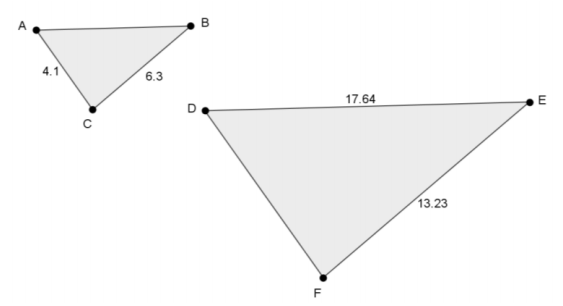1. Determine the length of side ${\overline{AB}}$
2. Determine the length of side ${\overline{DF}}$

#### References

EngageNY Mathematics Grade 8 Mathematics > Module 3 > Topic B > Lesson 11Teacher Version: Exit Ticket

Grade 8 Mathematics > Module 3 > Topic B > Lesson 11 of the New York State Common Core Mathematics Curriculum from EngageNY and Great Minds. © 2015 Great Minds. Licensed by EngageNY of the New York State Education Department under the CC BY-NC-SA 3.0 US license. Accessed Dec. 2, 2016, 5:15 p.m..

Modified by The Match Foundation, Inc.

?